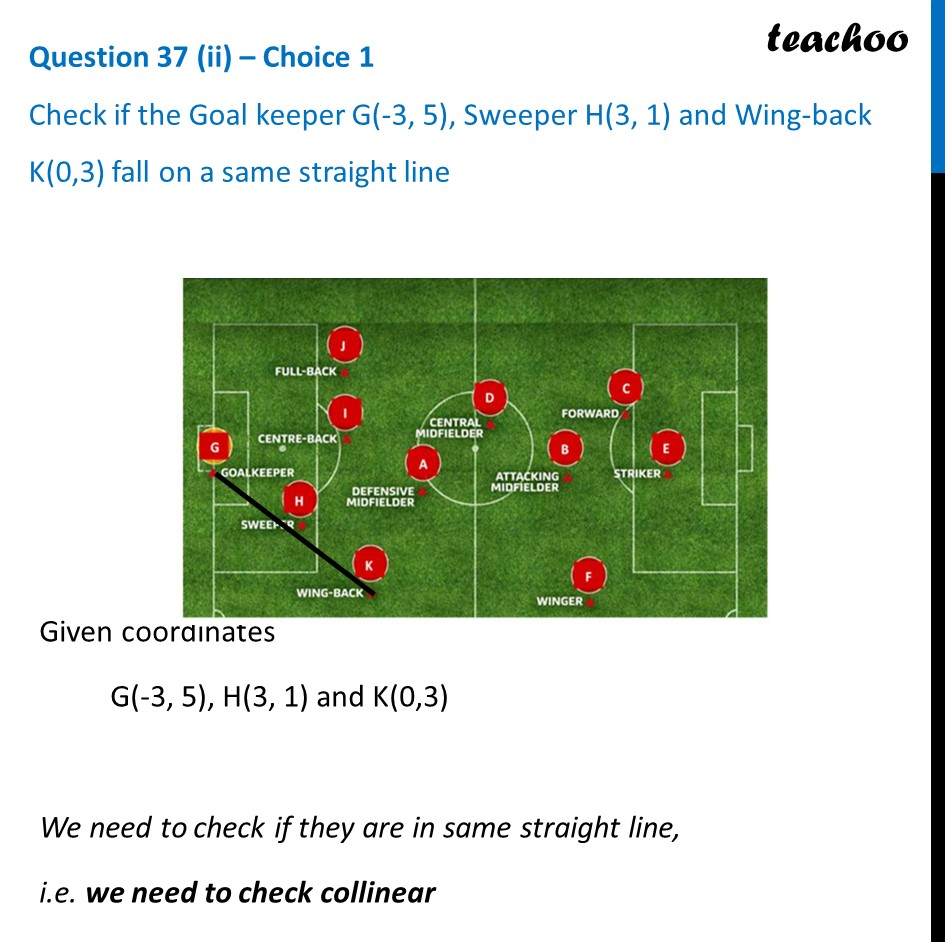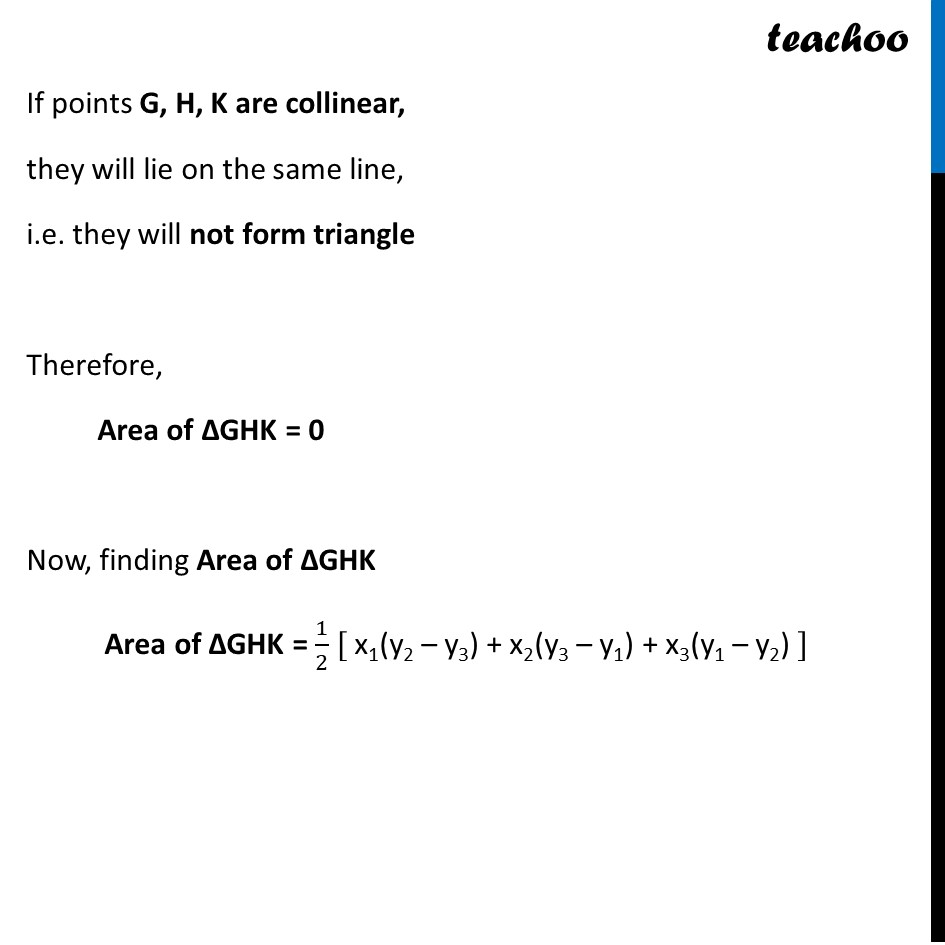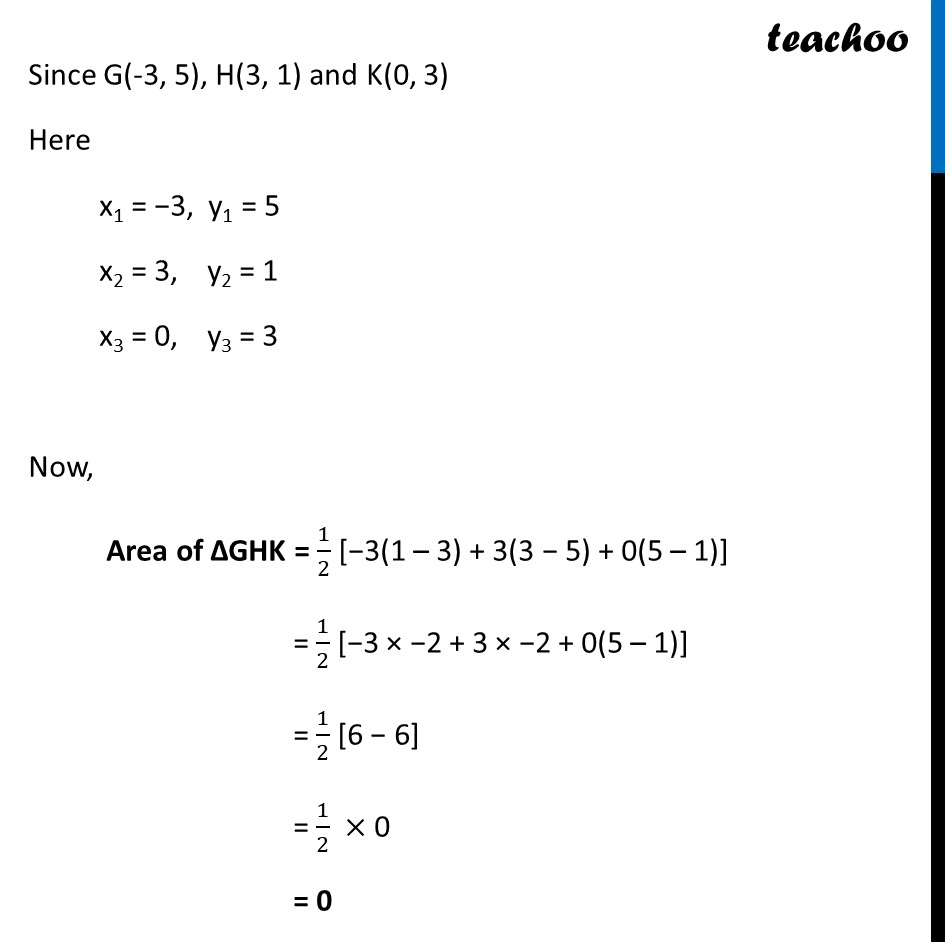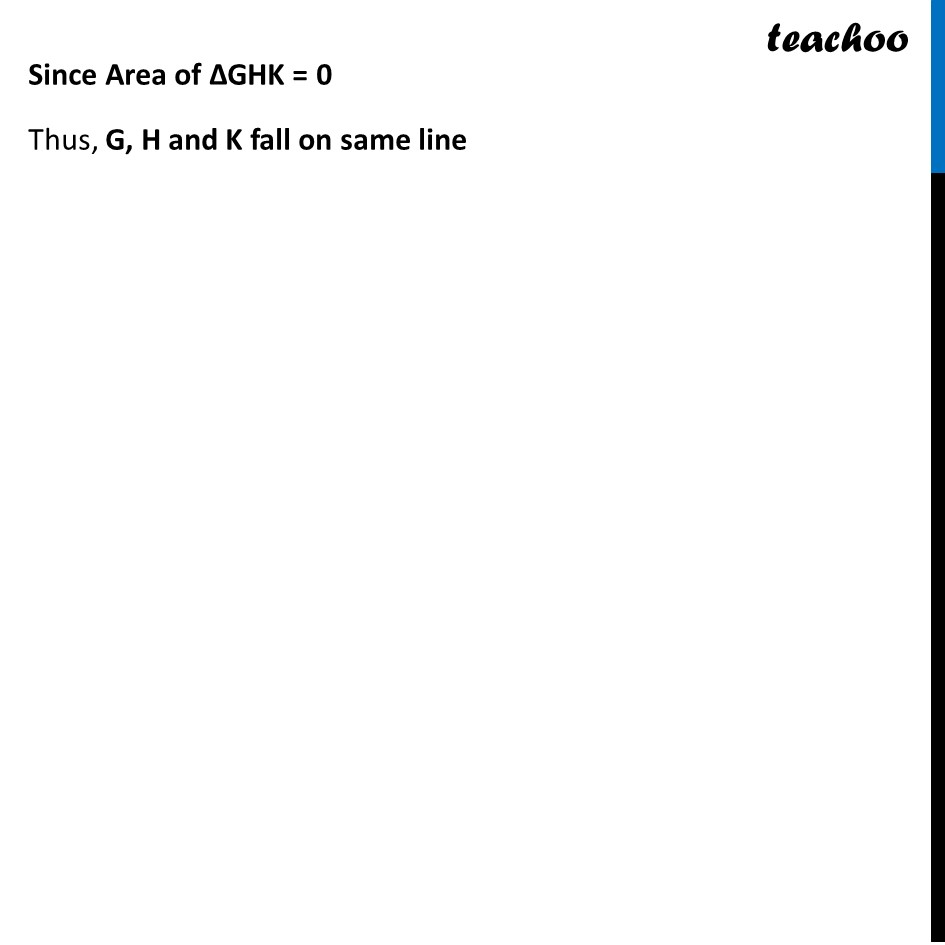CBSE Class 10 Sample Paper for 2024 Boards - Maths Standard

Class 10
Solutions of Sample Papers for Class 10 Boards

## Check if the Goal keeper G(-3, 5), Sweeper H(3, 1) and Wing-back K(0,3) fall on a same straight lineLearn in your speed, with individual attention - Teachoo Maths 1-on-1 Class

### Transcript

Given coordinates G(-3, 5), H(3, 1) and K(0,3) We need to check if they are in same straight line, i.e. we need to check collinear If points G, H, K are collinear, they will lie on the same line, i.e. they will not form triangle Therefore, Area of ∆GHK = 0 Now, finding Area of ∆GHK Area of ∆GHK = 1/2 [ x1(y2 – y3) + x2(y3 – y1) + x3(y1 – y2) ] Since G(-3, 5), H(3, 1) and K(0, 3) Here x1 = −3, y1 = 5 x2 = 3, y2 = 1 x3 = 0, y3 = 3 Now, Area of ∆GHK = 1/2 [−3(1 – 3) + 3(3 − 5) + 0(5 – 1)] = 1/2 [−3 × −2 + 3 × −2 + 0(5 – 1)] = 1/2 [6 − 6] = 1/2 × 0 = 0 Since Area of ∆GHK = 0 Thus, G, H and K fall on same line